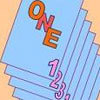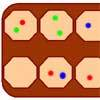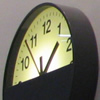Search by Topic

Resources tagged with Visualising similar to Partly Painted Cube:

Filter by: Content type:
Age range:
Challenge level:

There are 176 results

Broad Topics > Using, Applying and Reasoning about Mathematics > VisualisingPartly Painted Cube

Age 14 to 16 Challenge Level:

Jo made a cube from some smaller cubes, painted some of the faces of the large cube, and then took it apart again. 45 small cubes had no paint on them at all. How many small cubes did Jo use?Marbles in a Box

Age 11 to 16 Challenge Level:

How many winning lines can you make in a three-dimensional version of noughts and crosses?Painted Cube

Age 14 to 16 Challenge Level:

Imagine a large cube made from small red cubes being dropped into a pot of yellow paint. How many of the small cubes will have yellow paint on their faces?Building Gnomons

Age 14 to 16 Challenge Level:

Build gnomons that are related to the Fibonacci sequence and try to explain why this is possible.Jam

Age 14 to 16 Challenge Level:

A game for 2 playersTriangles Within Squares

Age 14 to 16 Challenge Level:

Can you find a rule which relates triangular numbers to square numbers?Steel Cables

Age 14 to 16 Challenge Level:

Some students have been working out the number of strands needed for different sizes of cable. Can you make sense of their solutions?Picturing Triangular Numbers

Age 11 to 14 Challenge Level:

Triangular numbers can be represented by a triangular array of squares. What do you notice about the sum of identical triangle numbers?Cubes Within Cubes Revisited

Age 11 to 14 Challenge Level:

Imagine starting with one yellow cube and covering it all over with a single layer of red cubes, and then covering that cube with a layer of blue cubes. How many red and blue cubes would you need?Triangles Within Triangles

Age 14 to 16 Challenge Level:

Can you find a rule which connects consecutive triangular numbers?One Out One Under

Age 14 to 16 Challenge Level:

Imagine a stack of numbered cards with one on top. Discard the top, put the next card to the bottom and repeat continuously. Can you predict the last card?Picturing Square Numbers

Age 11 to 14 Challenge Level:

Square numbers can be represented as the sum of consecutive odd numbers. What is the sum of 1 + 3 + ..... + 149 + 151 + 153?Hypotenuse Lattice Points

Age 14 to 16 Challenge Level:

The triangle OMN has vertices on the axes with whole number co-ordinates. How many points with whole number coordinates are there on the hypotenuse MN?Cogs

Age 11 to 14 Challenge Level:

A and B are two interlocking cogwheels having p teeth and q teeth respectively. One tooth on B is painted red. Find the values of p and q for which the red tooth on B contacts every gap on the. . . .Seven Squares - Group-worthy Task

Age 11 to 14 Challenge Level:

Choose a couple of the sequences. Try to picture how to make the next, and the next, and the next... Can you describe your reasoning?Konigsberg Plus

Age 11 to 14 Challenge Level:

Euler discussed whether or not it was possible to stroll around Koenigsberg crossing each of its seven bridges exactly once. Experiment with different numbers of islands and bridges.Sliding Puzzle

Age 11 to 16 Challenge Level:

The aim of the game is to slide the green square from the top right hand corner to the bottom left hand corner in the least number of moves.Khun Phaen Escapes to Freedom

Age 11 to 14 Challenge Level:

Slide the pieces to move Khun Phaen past all the guards into the position on the right from which he can escape to freedom.Tourism

Age 11 to 14 Challenge Level:

If you can copy a network without lifting your pen off the paper and without drawing any line twice, then it is traversable. Decide which of these diagrams are traversable.Building Tetrahedra

Age 14 to 16 Challenge Level:

Can you make a tetrahedron whose faces all have the same perimeter?Frogs

Age 11 to 14 Challenge Level:

How many moves does it take to swap over some red and blue frogs? Do you have a method?Jam

Age 14 to 16 Challenge Level:

To avoid losing think of another very well known game where the patterns of play are similar.A Tilted Square

Age 14 to 16 Challenge Level:

The opposite vertices of a square have coordinates (a,b) and (c,d). What are the coordinates of the other vertices?Christmas Chocolates

Age 11 to 14 Challenge Level:

How could Penny, Tom and Matthew work out how many chocolates there are in different sized boxes?Route to Infinity

Age 11 to 14 Challenge Level:

Can you describe this route to infinity? Where will the arrows take you next?Dotty Triangles

Age 11 to 14 Challenge Level:

Imagine an infinitely large sheet of square dotty paper on which you can draw triangles of any size you wish (providing each vertex is on a dot). What areas is it/is it not possible to draw?Hidden Rectangles

Age 11 to 14 Challenge Level:

Rectangles are considered different if they vary in size or have different locations. How many different rectangles can be drawn on a chessboard?Coloured Edges

Age 11 to 14 Challenge Level:

The whole set of tiles is used to make a square. This has a green and blue border. There are no green or blue tiles anywhere in the square except on this border. How many tiles are there in the set?Around and Back

Age 14 to 16 Challenge Level:

A cyclist and a runner start off simultaneously around a race track each going at a constant speed. The cyclist goes all the way around and then catches up with the runner. He then instantly turns. . . .Chess

Age 11 to 14 Challenge Level:

What would be the smallest number of moves needed to move a Knight from a chess set from one corner to the opposite corner of a 99 by 99 square board?Mystic Rose

Age 14 to 16 Challenge Level:

Use the animation to help you work out how many lines are needed to draw mystic roses of different sizes.Cuboid Challenge

Age 11 to 16 Challenge Level:

What's the largest volume of box you can make from a square of paper?There and Back Again

Age 11 to 14 Challenge Level:

Bilbo goes on an adventure, before arriving back home. Using the information given about his journey, can you work out where Bilbo lives?Take Ten

Age 11 to 14 Challenge Level:

Is it possible to remove ten unit cubes from a 3 by 3 by 3 cube so that the surface area of the remaining solid is the same as the surface area of the original?AMGM

Age 14 to 16 Challenge Level:

Can you use the diagram to prove the AM-GM inequality?An Unusual Shape

Age 11 to 14 Challenge Level:

Can you maximise the area available to a grazing goat?On the Edge

Age 11 to 14 Challenge Level:

If you move the tiles around, can you make squares with different coloured edges?Tetra Square

Age 11 to 14 Challenge Level:

ABCD is a regular tetrahedron and the points P, Q, R and S are the midpoints of the edges AB, BD, CD and CA. Prove that PQRS is a square.Tetrahedra Tester

Age 11 to 14 Challenge Level:

An irregular tetrahedron is composed of four different triangles. Can such a tetrahedron be constructed where the side lengths are 4, 5, 6, 7, 8 and 9 units of length?One and Three

Age 14 to 16 Challenge Level:

Two motorboats travelling up and down a lake at constant speeds leave opposite ends A and B at the same instant, passing each other, for the first time 600 metres from A, and on their return, 400. . . .Clocked

Age 11 to 14 Challenge Level:

Is it possible to rearrange the numbers 1,2......12 around a clock face in such a way that every two numbers in adjacent positions differ by any of 3, 4 or 5 hours?Tied Up

Age 14 to 16 Short Challenge Level:

How much of the field can the animals graze?Framed

Age 11 to 14 Challenge Level:

Seven small rectangular pictures have one inch wide frames. The frames are removed and the pictures are fitted together like a jigsaw to make a rectangle of length 12 inches. Find the dimensions of. . . .Age 11 to 14 Challenge Level:

Four rods, two of length a and two of length b, are linked to form a kite. The linkage is moveable so that the angles change. What is the maximum area of the kite?Flight of the Flibbins

Age 11 to 14 Challenge Level:

Blue Flibbins are so jealous of their red partners that they will not leave them on their own with any other bue Flibbin. What is the quickest way of getting the five pairs of Flibbins safely to. . . .Squares, Squares and More Squares

Age 11 to 14 Challenge Level:

Can you dissect a square into: 4, 7, 10, 13... other squares? 6, 9, 12, 15... other squares? 8, 11, 14... other squares?Wari

Age 14 to 16 Challenge Level:

This is a simple version of an ancient game played all over the world. It is also called Mancala. What tactics will increase your chances of winning?Rati-o

Age 11 to 14 Challenge Level:

Points P, Q, R and S each divide the sides AB, BC, CD and DA respectively in the ratio of 2 : 1. Join the points. What is the area of the parallelogram PQRS in relation to the original rectangle?Dice, Routes and Pathways

Age 5 to 14

This article for teachers discusses examples of problems in which there is no obvious method but in which children can be encouraged to think deeply about the context and extend their ability to. . . .Clocking Off

Age 7 to 16 Challenge Level:

I found these clocks in the Arts Centre at the University of Warwick intriguing - do they really need four clocks and what times would be ambiguous with only two or three of them?